### Formal Language & Automata Theory(New)

This is the online course on Formal Language & Automata Theory for Even Semester 2020 Batch. Due to COVID-19 Lockdown in the city of Kolkata, this becomes imperative for the students. Students are required to complete this course for their own benefit.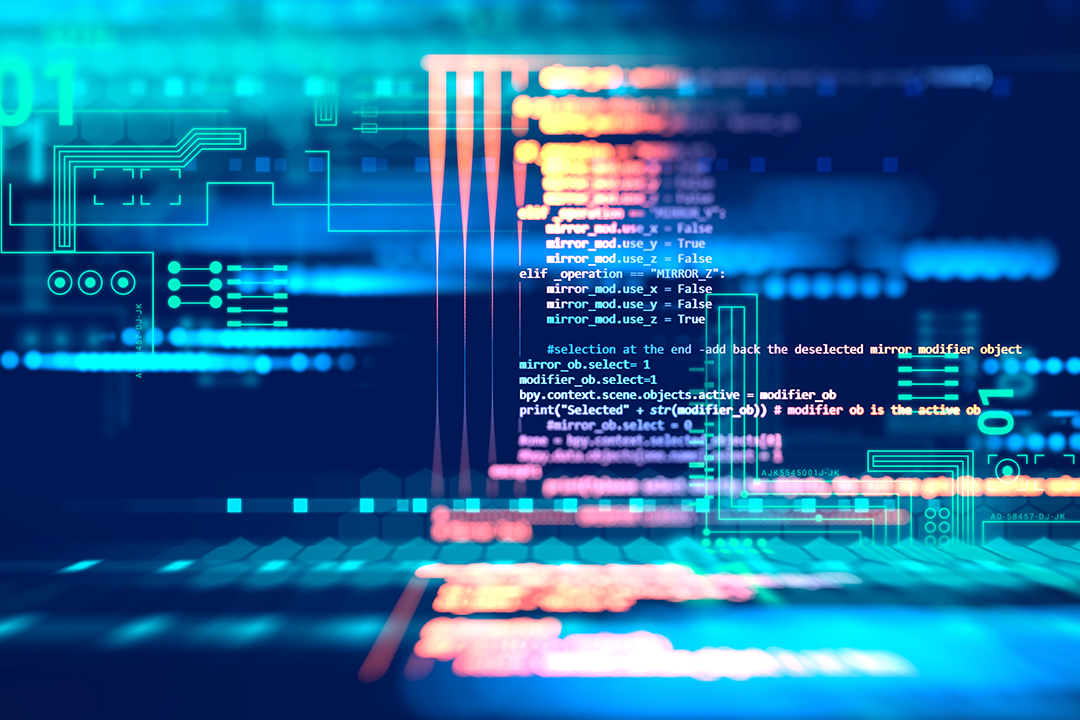### Project Management Training 2017-21 Batch### 2nd Year Domain Knowledge Training Online Test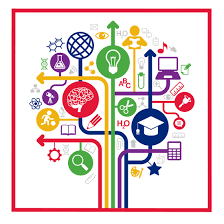### Software Tools

Course Objective

Design, create and debug windows applications using Visual Basic programming.

ii)   Course Outcomes

After completion of this course the students will be able to

1.      Identify the differences between the procedural languages and event-driven languages and understanding how a windows program runs and also Load, modify, and save changes made to forms and projects in the Visual Basic environment.

2.      Implement Windows based application using Visual Basic, sub procedures, Functions, forms, and Windows environment calls.

3.      Define and implement form objects including data arrays, control arrays, and different types of control like text boxes, message boxes, dialog boxes, labels, controls, menus, frames, picture boxes, pull-down menus, and combo boxes, timer control, etc.

4.      Understand and apply MDI forms, database handling using form with various controls with ODBC connectivity.

5.      Case Studies. e, g, Student Management System, Hospital Management System, Geographical information system, etc.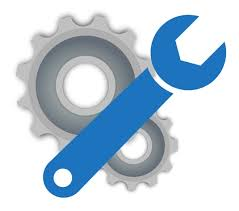### Second Year Non-Departmental Course Feedback### Computer Architecture

Objective:

1 To learn the basics of stored program concepts.

2 To learn the principles of pipelining.

3 To learn mechanism of data storage.

4 To distinguish between the concepts of serial, parallel, pipeline architecture.

Course Outcomes:

On completion of the course students will be able to

1 Learn pipelining concepts with a prior knowledge of stored program methods.

2 Learn about memory hierarchy and mapping techniques.

3 Study of parallel architecture and interconnection network.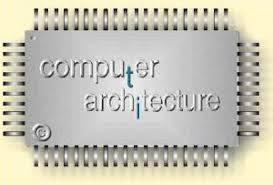### Data Structure & Algorithm

Course Objective:  Students are expected to be able to learn various data structures, its different operations and fundamental algorithms.

Course Outcomes: After completion of this course the students are expected to be able to demonstrate following knowledge, skills and attitudes

1. Derive Time and Space properties of data structures and basic algorithm in terms of Time and Space complexity.
2. Compare the advantages and drawbacks of different data structures and how their drawbacks can be overcome.
3. Identify the appropriate data structure to implement a basic algorithm.
4. Ability to demonstrate various operations on different linear and nonlinear data structure using C language such as array, stacks, queues, linked lists, binary tree etc.
5. Survey the different application areas of different data structures.### Design & Analysis of Algorithm

Course Objective:

To explain the concept of asymptotic complexity with different classes of problems and solution strategies available, and finally evaluate by comparing them.

Course Outcomes:

After completion of this course the students are expected to be able to demonstrate following knowledge, skills and attitudes :-

1.For a given algorithm analyze the worst-case running times, of algorithms based on asymptotic analysis and justify the correctness of the algorithm.

2. Describe the greedy paradigm and explain when an algorithmic design situation calls for it. For a given problem develop the greedy algorithms.

3. Describe the divide-and-conquer paradigm and explain when an algorithmic design situation calls for it. Synthesize divide-and-conquer algorithms. Derive and solve recurrence relation.

4. Describe the dynamic-programming paradigm and explain when an algorithmic design situation calls for it. For a given problems of dynamic-programming .

5. Develop the dynamic programming algorithms, and analyze it to determine its computational complexity.

6. For a given model engineering problem model it using graph and write the corresponding algorithm to solve the problems.

7. Explain the ways to analyze randomized algorithms (expected running time, probability of error).

8. Explain what an approximation algorithm is. Compute the approximation factor of an approximation algorithm (PTAS and FPTAS).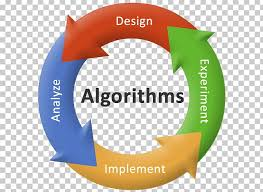### Computer Organization

Course Description: This course is a study of the evolution of computer architecture and the factors influencing the design of hardware and software elements of computer systems. We will engage in a detailed, bottom-up study of the major hardware components of a basic computer system. This study includes logic gates, combinational circuits, circuits for arithmetic, simple arithmetic logic unit for a particular machine, memory structures, the data path and the control unit and I/O units.

Course Objective:To identify the attributes, functionalities and organizational structure of a computer system by deconstructing it into the salient components.

Course Outcome:

1. Identify and describe the basic digital components, various data representations, arithmetic and logical operations performed by computers.
2. Determine the steps in the execution of a computer operation, summarizing the role of the components.
3. Implement various modules of a computer system using the knowledge of the basic digital components.
4. Explain and analyze the function and interplay of various components in a computer.
5. Compare the impact of different technological changes on computer design and their relative merits.